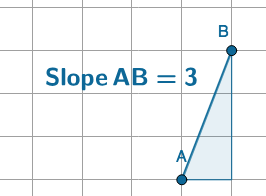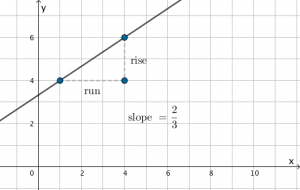# The slope of a line

The slope of a line tells us how steep the line is. For one unit to the right, how much increase is made vertically?

Here is a line segment with a slope of 3. One unit horizontally, 3 units vertically.Here is a line segment with a slope of a half. One unit horizontally, half a unit vertically. It is easier to draw if you count two units horizontally, 1 unit vertically.It is often calculated asA horizontal line has slope 0. There is no increase or decrease.

A line at 45° has a slope of 1. That is, one unit to the right, one unit up.

Move the slider to see slope values between zero and one.

A line that has slope more than one is quite steep.

A line with negative slope is a line that goes down to the right.

Use the applet to create line segments of the following slopes:

1. Slope AB = 5
2. SlopeAB = -3
3. Slope AB = 0
4. Slope AB = 1
5. Slope AB = -1
6. Infinitely steep (vertical)
7. Slope AB =8. Slope AB =# Test out: Skill 2

Get 8 correct to test out of this skill.

fg